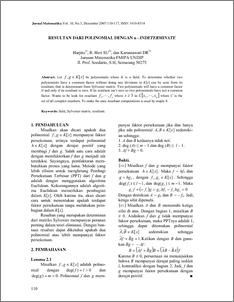# RESULTAN DARI POLINOMIAL DENGAN n - INDETERMINATE

Harjito, Harjito and R. Heri SU, R. Heri SU and Karuniawati DR, Karuniawati DR (2007) RESULTAN DARI POLINOMIAL DENGAN n - INDETERMINATE. JURNAL MATEMATIKA MATEMATIKA, 10 (3). pp. 110-117. ISSN 1410-8518Preview
PDF
202Kb

## Abstract

Let be polynomials where K is a field. To determine whether two polynomials have a common factor without doing any divisions in K[x] can be seen from its resultant, that is determinant from Sylvester matrix. Two polynomials will have a common factor if and only if its resultant is zero. If its resultant isn’t zero so two polynomials have not a common factor. Wants to be look for resultant where in where C is the set of all complex numbers. To make the easy resultant computations is used by maple 8.

Item Type: Article field, Sylvester matrix, resultant Q Science > Q Science (General)Q Science > QA Mathematics Faculty of Science and Mathematics > Department of Mathematics 1870 INVALID USER 30 Nov 2009 11:39 08 Dec 2009 09:14

Repository Staff Only: item control page